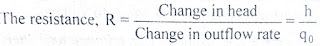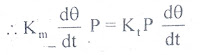# Transfer Function of Hydraulic System

0
878

## Transfer Function & Mathematical Model of Hydraulic System:

#### Consider the flow through a short pipe connecting two tanks. The Resistance, R for liquid flow in such a pipe or restriction, is defined as the change in the level difference, necessary to cause a unit change in the flow rate.#### The Capacitance, C of a tank is defined to he the change in quantity of stored liquid necessary to cause in the potential (head).### Example of Liquid Level System:

#### A simple liquid level system is shown in the below figure with steady flow rate, Q and steady state head,H.#### Let     Q = Steady state flow rate( before any change has occured) qi = Small deviation of inflow rate from its steady-state value qo = Small deviation of outflow rate from its steady-state value H = Steady state head (before any change has occured) h = Small deviation of head from its steady state valueLet the system  be considered linear.The differential equation governing the system is obtained by equating the change in flow rate to the amount stored in the tank.In a small time interval dt, let the change in flow rate be (qi – qo) and the change in height be dh.Now, Change In storage = Change in flow rate C dh = (qi – qo) dhqo = h/R

#### RC dh/dt + h = qi RThe above equation is the differential equation governing the system.The term RC is the time constant of the system .On the Laplace’s transform of above equation we get,### Transfer function of Hydraulic System:

#### The most frequently used hydraulic device in control system is hydraulic motor-pump set.It consists of a variable stroke hydraulic pump and a fixed stroke hydraulic motor as shown in the below figure.The device accepts a linear displacement (stroke length) input and delivers a large output torque.Let us now derive the transfer function of hydraulic system.#### The hydraulic motor is controlled by the amount of oil delivered by the pump.By mechanically changing the pump stroke, the oil delivered by the pump is controlled.Like in a DC generator and dc motor, there is no essential difference between hydraulic pump and motor.In a pump the input is mechanical power and output is hydraulic power and in a motor, it is vice versa.Let         qp = Rate at which the oil flows from the pump qm = Oil flow rate through the motor q = Leakage flow rate qc = compressibility flow rate x = Input stroke length θ = Output angular displacement of motor P = Pressure drop across motor

The rate at which the oil flow from the pump is proportional to stroke angle, i.e., qp ∝ x.

Oil flow rate from-the pump, qp = Kp x
where Kp = Ratio of rate of oil flow to unit stroke angle.

The rate of oil flow through the motor is proportional to motor speed, i.e., qm ∝ dθ/dt.
Oil flow rate through motor, qm = Km dt

where Km = Motor displacement constant.

All the oil from the pump does not flow through the motor in the proper channels. Due to back pressure in the motor, a portion of the ideal flow from the pump leaks back past the pistons of motor and rump. The back pressure is the pressure that is built up by the hydraulic flow to overcome the resistance 😮 free movement offered by load on motor shaft.

It is usually assumed that the leakage flow is proportional to motor pressure, i.e. qi ∝ P
Leakage flow rate, qi = Ki P
where Ki = constant.
The back pressure built up by the motor not only causes leakage flow in the motor and pump but oil in the lines to compress.Volume compressibility flow is essentially proportional to pressure and therefore the rate of flow is proportional to the rate of change of pressure, i.e. qc ∝ dP/dt

Compressibility flow rate, qc = Kc dP/dt
where Kc = Coefficient of compressibility.

The rate at which the oil flows from the pump is given by sum of oil flow rate through the motor, leakage flow rate and compressibility flow rate.

qp = qm + qi + qc

On substituting from above equations,we getThe torque Tm developed by the motor is proportional to pressure drop and balances load torque.

Hydraulic motor torque, Tm = Kt P
where Kt is motor torque constant.

If the load is assumed to consist of moment of inertia J and viscous friction with coefficient B, then,On substituting for qm, we get,On substituting for Tm, we get,If hydraulic motor losses are neglected or include-d as a part of load, then the hydraulic motor input is equal to mechanical power output of hydraulic motor.From equation, it is clear that Km = Kt .

Hence  we can write,

Tm = Kt P = Km P

Since the motor torque equals load torque, Tm = Tl#### On differentiating above equation w.r.t ,we get,#### On substituting for P and dP/dt ,we get,#### On taking Laplace transform with zero initial conditions , we get,#### In hydraulic systems,normally  Kc << Km , therefore ,Put Kc = 0  in above equation.#### The above equation is the required transfer function of hydraulic system.

READ HERE  Transfer Function of Field Controlled DC Motor